# Mehler-Fock transform

(Redirected from Mehler–Fock transform)

Mehler–Fok transform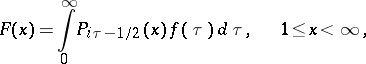(1)

where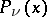is the Legendre function of the first kind (cf. Legendre functions). If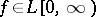, the function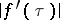is locally integrable on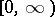and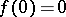, then the following inversion formula is valid:(2)

The Parseval identity. Consider the Mehler–Fock transform and its inverse defined by the equalities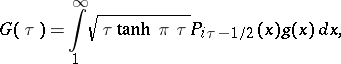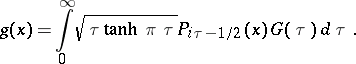If,, are arbitrary real-valued functions satisfying the conditionsthenThe generalized Mehler–Fock transform and the corresponding inversion formula are:(3)

and(4)where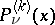are the associated Legendre functions of the first kind. For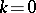formulas (3) and (4) reduce to (1) and (2); for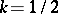,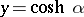, formulas (3) and (4) lead to the Fourier cosine transform, and for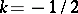,to the Fourier sine transform. The transforms (1) and (2) were introduced by F.G. Mehler . The basic theorems were proved by V.A. Fock [V.A. Fok].